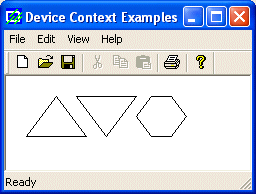YOU CAN CODE!With The Case Of UCanCode.net  Release The Power OF  Visual C++ ! Home |  Products | Purchase |  Support | Downloads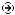Download EvaluationPricing & Purchase?E-XD++Visual C++/ MFC ProductsOverviewElectronic Form SolutionVisualization & HMI SolutionPower system HMI SolutionBar code labeling SolutionWorkflow SolutionCoal industry HMI SolutionInstrumentation Gauge SolutionReport Printing SolutionGraphical modeling SolutionGIS mapping solutionVisio graphics solutionIndustrial monitoring SolutionFlowchart and diagramming SolutionOrganization Diagram SolutionGraphic editor Source CodeUML drawing editor Source CodeMap Diagramming SolutionArchitectural Graphic Drawing SolutionRequest EvaluationActiveX COM ProductsOverviewTechnical Support# Get Ready to Unleash the Power of UCanCode .NET

UCanCode Software focuses on general application software development. We provide complete solution for developers. No matter you want to develop a simple database workflow application, or an large flow/diagram based system, our product will provide a complete solution for you. Our product had been used by hundreds of top companies around the world!

"100% source code provided! Free you from not daring to use components because of unable to master the key technology of components!"

 GDI Topics:  PolyPolyline, Polyline, PolylineTo

 Introduction
 A polyline is a series of connected lines. The lines are stored in an array of POINT or CPoint values. To draw a polyline, you use the CDC::Polyline() method. Its syntax is: `BOOL Polyline(LPPOINT lpPoints, int nCount);` The lpPoints argument is an array of points that can be of POINT or CPoint types. The nCount argument specifies the number of members of the array. When executing, the compiler moves the starting point to lpPoints. The first line is drawn from lpPoints to lpPoints as in:
```void CExoView::OnDraw(CDC* pDC)
{
CPoint Pt[] = { CPoint(60, 20), CPoint(60, 122) };
pDC->MoveTo(Pt);
pDC->LineTo(Pt);
}```

To draw a polyline, you must have at least two points. If you define more than two points, each line after the first would be drawn from the previous point to the next point until all points have been included. Here is an example:

 ```void CExoView::OnDraw(CDC* pDC) { CPoint Pt; Pt = CPoint(20, 50); Pt = CPoint(180, 50); Pt = CPoint(180, 20); Pt = CPoint(230, 70); Pt = CPoint(180, 120); Pt = CPoint(180, 90); Pt = CPoint(20, 90); pDC->Polyline(Pt, 7); }```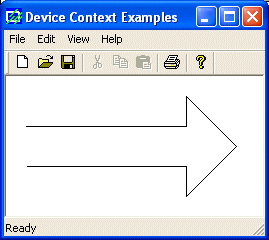Besides the Polyline() method, the CDC class provides the PolylineTo() member function. Its syntax is:

`BOOL PolylineTo(const POINT* lpPoints, int nCount);`

The lpPoints argument is the name of an array of POINT or CPoint objects. The nCount argument specifies the number of points that would be included in the figure. Here is an example:

 ```void CExoView::OnDraw(CDC* pDC) { CPoint Pt; Pt = CPoint(20, 50); Pt = CPoint(180, 50); Pt = CPoint(180, 20); Pt = CPoint(230, 70); Pt = CPoint(180, 120); Pt = CPoint(180, 90); Pt = CPoint(20, 90); pDC->PolylineTo(Pt, 7); }```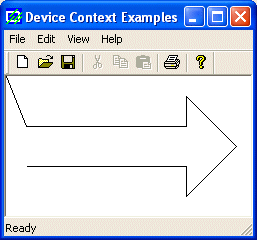While the Polyline() method starts the first line at lpPoints, the PolylineTo() member function does not control the beginning of the first line. Like the LineTo() method, it simply starts drawing, which would mean it starts at the origin (0, 0). For this reason, if you want to control the starting point of the PolylineTo() drawing, you can use the MoveTo() method:

 ```void CExoView::OnDraw(CDC* pDC) { CPoint Pt; Pt = CPoint(20, 50); Pt = CPoint(180, 50); Pt = CPoint(180, 20); Pt = CPoint(230, 70); Pt = CPoint(180, 120); Pt = CPoint(180, 90); Pt = CPoint(20, 90); pDC->MoveTo(20, 30); pDC->PolylineTo(Pt, 7); pDC->LineTo(20, 110); }```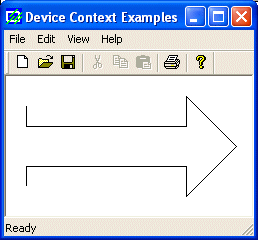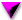Practical Learning:  Drawing Polylines
1. To draw a polyline, change the event as follows:

 ```void CView1View::OnPaint() { CPaintDC dc(this); // device context for painting // TODO: Add your message handler code here CPoint PtLine[] = { CPoint( 50, 50), CPoint(670, 50), CPoint(670, 310), CPoint(490, 310), CPoint(490, 390), CPoint(220, 390), CPoint(220, 310), CPoint( 50, 310), CPoint( 50, 50) }; CPoint PlLine[] = { CPoint( 55, 55), CPoint(665, 55), CPoint(665, 305), CPoint(485, 305), CPoint(485, 385), CPoint(225, 385), CPoint(225, 305), CPoint( 55, 305), CPoint(55, 55) }; dc.MoveTo(PtLine); dc.LineTo(PtLine); dc.LineTo(PtLine); dc.LineTo(PtLine); dc.LineTo(PtLine); dc.LineTo(PtLine); dc.LineTo(PtLine); dc.LineTo(PtLine); dc.LineTo(PtLine); dc.Polyline(PlLine, 9); // Do not call CView::OnPaint() for painting messages }```
2. Test the application
 Multiple Polylines

The above polylines were used each as a single entity. That is, a polyline is a combination of lines. If you want to draw various polylines in one step, you can use the CDC::PolyPolyline() method. By definition, the PolyPolyline() member function is used to draw a series of polylines. Its syntax is:

`BOOL PolyPolyline(const POINT* lpPoints, const DWORD* lpPolyPoints, int nCount);`

Like the above Polyline() method, the lpPoints argument is an array of POINT or CPoint values. The PolyPolyline() method needs to know how many polylines you would be drawing.  Each polyline will use the points of the lpPoints value but when creating the array of points, the values must be incremental. This means that PolyPolyline() will not access their values at random. It will retrieve the first point, followed by the second, followed by the third, etc. Therefore, your first responsibility is to decide where one polyline starts and where it ends. The good news (of course depending on how you see it) is that a polyline does not start where the previous line ended. Each polyline has its own beginning and its own ending point.

Unlike Polyline(), here, the nCount argument is actually the number of shapes you want to draw and not the number of points (remember that each polyline "knows" or controls its beginning and end).

The lpPolyPoints argument is an array or positive integers (unsigned long). Each member of this array specifies the number of vertices (lines) that its corresponding polyline will have. For example, imagine you want to draw M, followed by L, followed by Z. The letter M has 4 lines but you need 5 points to draw it. The letter L has 2 lines and you need 3 points to draw it. The letter Z has 3 lines so 4 points are necessary to draw it. You can store this combination of lines in an array defined as { 5, 3, 4 }.

Here is an example:

 ```void CExoView::OnDraw(CDC* pDC) { CPoint Pt; DWORD lpPts[] = { 4, 4, 7 }; // Left Triangle Pt = Pt = CPoint(50, 20); Pt = CPoint(20, 60); Pt = CPoint(80, 60); // Second Triangle Pt = Pt = CPoint(70, 20); Pt = CPoint(100, 60); Pt = CPoint(130, 20); // Hexagon Pt = Pt = CPoint(145, 20); Pt = CPoint(130, 40); Pt = CPoint(145, 60); Pt = CPoint(165, 60); Pt = CPoint(180, 40); Pt = CPoint(165, 20); pDC->PolyPolyline(Pt, lpPts, 3); }```